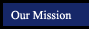Section Page Segment Measure and Coordinate Graphing 2.1 50 Real Numbers and Number Lines vocabulary whole numbers, natural (or counting) numbers, integers, rational numbers, terminating decimals, non-terminating decimals, irrational numbers, real numbers, coordinate, origin, absolute value theorem/postulates Postulate 2-1, Postulate 2-2, Postulate 2-3 2.2 56 Segments and Properties of Real Numbers new per2 per6 honors class - per 5 vocabulary betweenness, equation, measurement, unit of measure, precision, greatest possible error, percent of error theorem/postulates reflexive property, symmetric property, transitive property, addition and subtraction properties, multiplication and division properties, substitution property 2.3 62 Congruent Segments new per2 per6 honors class - per 5 vocabulary congruent segments, theorem, midpoint, bisect construction segment bisector (a.k.a. perpendicular bisector) theorem/postulates Theorem 2-1, Theorem 2-2, Theorem 2-3 2.4 68 The Coordinate Plane new per2 per6 honors class - per 5 vocabulary coordinate plane, x-axis, y-axis, quadrant, origin, ordered pair, coordinates, graph, x-coordinate, y-coordinate theorem/postulates Theorem 2-4 2.5 76 Midpoints new per2 per6 honors class - per 5 vocabulary no new vocabulary theorem/postulates Theorem 2-5, Theorem 2-6